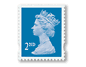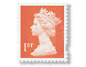#### You may also like### Exploring Wild & Wonderful Number Patterns

EWWNP means Exploring Wild and Wonderful Number Patterns Created by Yourself! Investigate what happens if we create number patterns using some simple rules.### Sending Cards

This challenge asks you to investigate the total number of cards that would be sent if four children send one to all three others. How many would be sent if there were five children? Six?### Dice and Spinner Numbers

If you had any number of ordinary dice, what are the possible ways of making their totals 6? What would the product of the dice be each time?

# Penta Post

##### Age 7 to 11Challenge Level

Here are the prices for 1st and 2nd class mail within the UK [in 2002].

Weight up to First Class Second Class
$60g$ $27p$ $19p$
$100g$ $41p$ $33p$
$150g$ $57p$ $44p$
$200g$ $72p$ $54p$
$250g$ $84p$ $66p$
$300g$ $96p$ $76p$
$350g$ $£1.09$ $87p$
$400g$ $£1.30$ $£1.05$
$450g$ $£1.48$ $£1.19$
$500g$ $£1.66$ $£1.35$
$600g$ $£2.00$ $£1.60$
$700g$ $£2.51$ $£1.83$
$750g$ $£2.69$ $£1.94$*
$800g$ $£2.91$
$900g$ $£3.20$
$1kg$ $£3.49$

Costs for First Class items over $1kg$ are $£3.49$ and then $85p$ for each extra $250g$.
*Items over $750g$ cannot be sent second class.

You have an unlimited number of each of these stamps:$4p$ $10p$ $19p$ $27p$ $37p$ $£1.00$

1/ Which stamps would you need to post a parcel weighing $825g$?

2/ I want to send a package 1st class which weighs $235g$. It is very small so I want to use as few stamps as possible. Which ones would I use?

3/ If I only had $3$ of each kind of stamp, which $2$nd class price could I not make?

4/ How many different combinations of stamps could be stuck on a letter weighing $140g$ if it goes 1st class?

5/ I use the following stamps to send two items, one 1st class and the other 2nd class:What could their weights be?

Further extension to this activity can be carried out by considering the value of the stamps alone, as numbers $4$, $10$, $19$, $27$, $37$ & $100$. For example taking the $5$ lowest numbers [missing out the $100$] challenging the pupils to come up with the smallest number of ways you can get totals between $4$ and $50$. This could lead to questions about what totals can NOT be had, and checking to see if you've really got the smallest number of ways, each time.